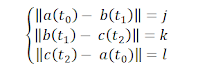## Thursday, August 26, 2010

### Projector calibration

In order to project a 3d scene on a real life environment it is necessary to match the virtual camera position with the one of the projector.

To do this there are many existing techniques using cameras and computer vision methods.
However, we developed a method that doesn't require the use of a camera.

The goal of this method is to obtain the position and orientation of the projector using a center of coordinates relative to the scene.
This problem is similar to finding the position and orientation of the chosen center of coordinates relative to the projector.

This method uses three known perpendicular points at the corners of the calibration surface that are aligned with the target coordinate system. Hence the distances between those points are known.

The projector casts rays of light and three of these rays pass through the chosen points and also through the center of the original coordinates system (inside the projector).
To obtain the equation of these rays only one point different from the origin is needed.
This point can be obtained from the screen coordinates of the pixel that is projected on each target point, for example, by clicking on them.

Using the screen coordinates of the point and some known projector parameters like the resolution and the projection angle it is possible to find the 3D coordinates of the point and then the equation of the ray.
Now that the equations for the three rays are known, we need to find the parameter t where the ray meets the corner of the target area. This can be written as a system of equations using the ray formula and the dimensions of the target area.a, b, c are the rays. j, k, l are the width, height and diagonal of the surface
The goal is to find the parameters t0, t1 and t2 that satisfy the non-linear system.
An approximation for this solution can be obtained using numerical methods. At the moment our implementation uses the trust-region-dogleg method from Matlab.

Once the values for t0, t1 and t2 are known, the coordinates for the corners of the target area are a(t0), b(t1) and c(t2). The base for the target coordinate system is

The location of the projector relative to this base of coordinates is obtained by projecting a(t0) on this base.